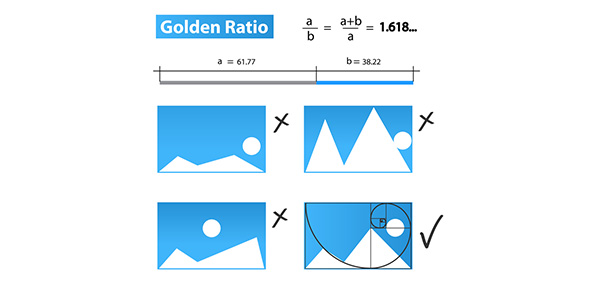# Trigonometric Ratios Quiz-2

18 QuestionsSettingsStudents should be able to find sine, cosine, tangent in a right triangle given 3 side lengths. They should also remember the side ratios of the special triangles and find s/c/t. They should also be able to identify opposite and adjacent sides.

Related Topics
• 1.
Find sin(A).
• A.

7/25

• B.

• C.

24/25

• 2.
Find tan(B).
• A.

• B.

7/24

• C.

7/25

• 3.
Find cos(B).
• A.

24/25

• B.

7/25

• C.

• 4.
Find sin(D).
• A.

12/13

• B.

5/13

• C.

12/5

• 5.
Find tan(E).
• A.

12/5

• B.

12/13

• C.

5/12

• 6.
• A.

AC

• B.

BC

• C.

AB

• 7.
Which side is opposite angle D?
• A.

DF

• B.

ED

• C.

EF

• 8.
Which LEG is adjacent to angle B?
• A.

BC

• B.

AC

• C.

AB

• 9.
Find tan(45).
• A.

1

• B.

Sqrt(2)

• C.

1/sqrt(2)

• 10.
Find cos(45).
• A.

1

• B.

Sqrt(2)

• C.

1/sqrt(2)

• 11.
Sin(45) = cos(45)
• A.

True

• B.

False

• 12.
You need a calculator to find tan(45).
• A.

True

• B.

False

• 13.
Find cos(60).
• A.

Sqrt(3)/2

• B.

1/2

• C.

Sqrt(3)

• 14.
Find tan(30).
• A.

Sqrt(3)/2

• B.

1/2

• C.

1/sqrt(3)

• 15.
Find sin(60).
• A.

Sqrt(3)/2

• B.

1/2

• C.

Sqrt(3)

• 16.
Sin(30) = cos(30)
• A.

True

• B.

False

• 17.
Sin(30) = cos(60)
• A.

True

• B.

False

• 18.
You need a calculator to find cos(60).
• A.

True

• B.

False